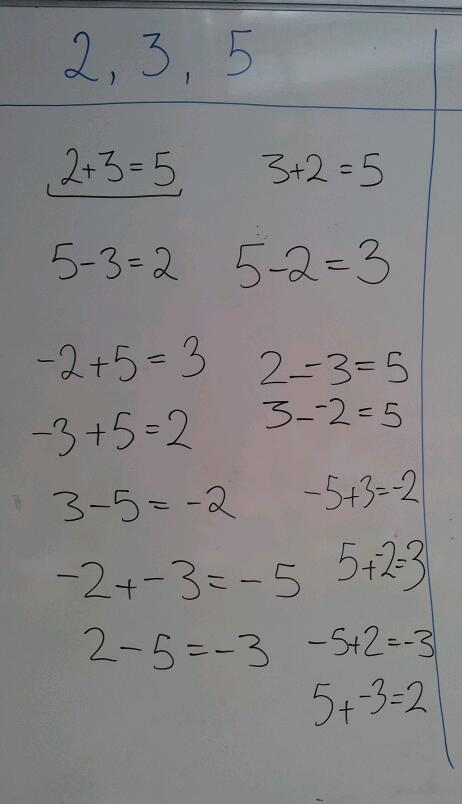# 224. No Nonsense Negatives

Ever had a simple idea for a starter which your class just flies with? It happened today for me:

Background
In the previous lesson students understood the meaning of ‘y=mx+c’, but struggled to rearrange equations in this form. With this in mind, I went back to the basics of manipulating calculations.

Starter question 1
Make as many calculations as you can only using the numbers 2, 3 & 5 (once each) and any symbol you like. The obvious answer is 2+3=5.

Starter question 2
Make as many calculations as you can only using the numbers 3, 6 & 18 (once each) and any symbol you like. The obvious answer is 3×6=18.

The Extension
Most groups quickly found three solutions for each question. Some even used inequalities. To extend their understanding I suggested that they could use as many of each symbol as they wished – would a sprinkling of minus signs increase the number of results?

Results
The following pictures show the ideas my class came up with. I was using lolly sticks to randomly pick students and no one wanted to be the first to not give an answer.We discussed the rearrangements and linked them to rearranging equations. They appreciated that one equation could be written in many different ways. This activity would work equally well to consolidate negative numbers.